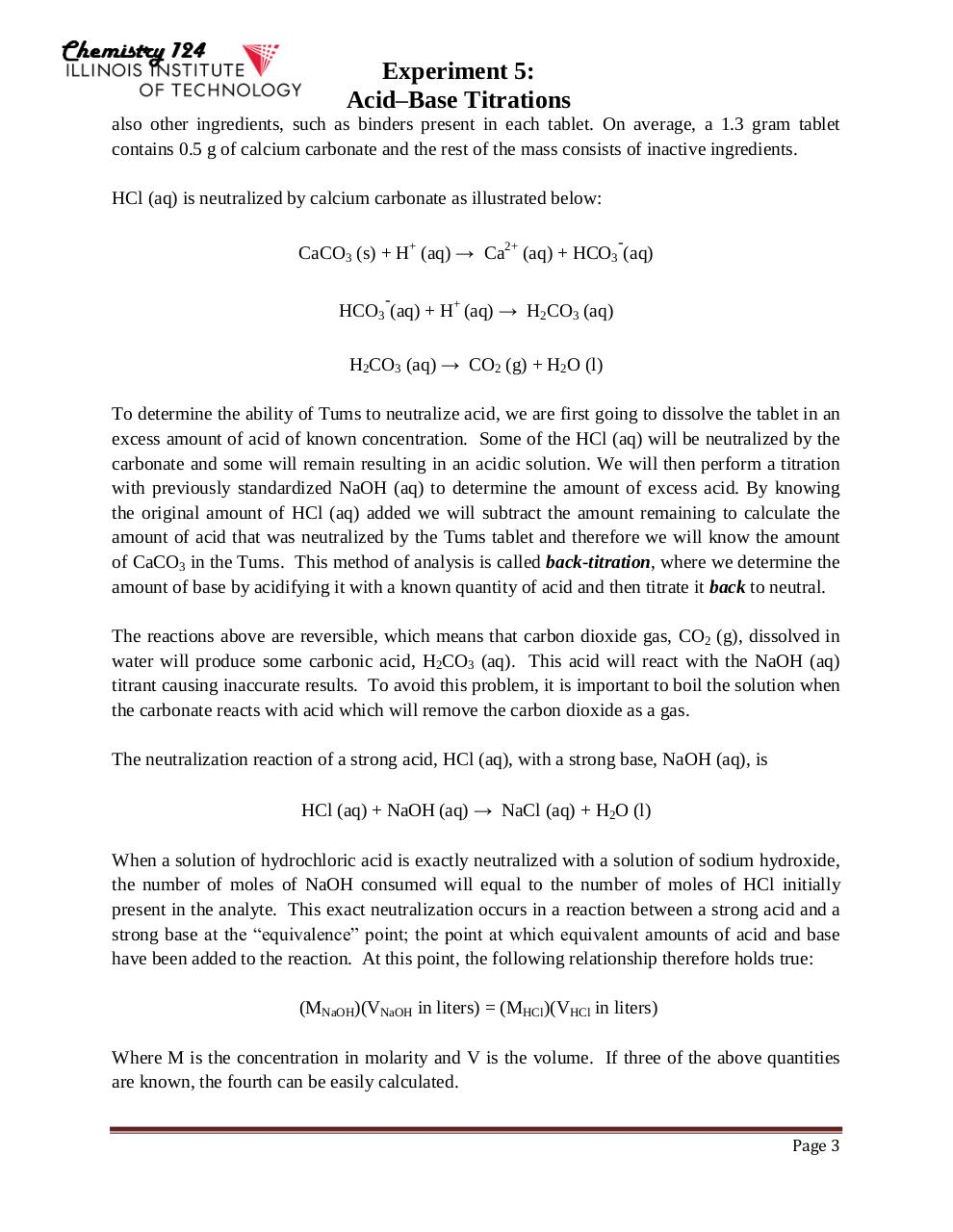# Lab 5 Titrations Fall 2015(1).pdfPage 1 2 3 4 5 6 7 8 9 10 11 12 13

#### Text preview

Experiment 5:
Acid–Base Titrations
also other ingredients, such as binders present in each tablet. On average, a 1.3 gram tablet
contains 0.5 g of calcium carbonate and the rest of the mass consists of inactive ingredients.
HCl (aq) is neutralized by calcium carbonate as illustrated below:
-

CaCO3 (s) + H+ (aq)→ Ca2+ (aq) + HCO3 (aq)
-

HCO3 (aq) + H+ (aq)→ H2CO3 (aq)
H2CO3 (aq)→ CO2 (g) + H2O (l)
To determine the ability of Tums to neutralize acid, we are first going to dissolve the tablet in an
excess amount of acid of known concentration. Some of the HCl (aq) will be neutralized by the
carbonate and some will remain resulting in an acidic solution. We will then perform a titration
with previously standardized NaOH (aq) to determine the amount of excess acid. By knowing
the original amount of HCl (aq) added we will subtract the amount remaining to calculate the
amount of acid that was neutralized by the Tums tablet and therefore we will know the amount
of CaCO3 in the Tums. This method of analysis is called back-titration, where we determine the
amount of base by acidifying it with a known quantity of acid and then titrate it back to neutral.
The reactions above are reversible, which means that carbon dioxide gas, CO2 (g), dissolved in
water will produce some carbonic acid, H2CO3 (aq). This acid will react with the NaOH (aq)
titrant causing inaccurate results. To avoid this problem, it is important to boil the solution when
the carbonate reacts with acid which will remove the carbon dioxide as a gas.
The neutralization reaction of a strong acid, HCl (aq), with a strong base, NaOH (aq), is
HCl (aq) + NaOH (aq)→ NaCl (aq) + H2O (l)
When a solution of hydrochloric acid is exactly neutralized with a solution of sodium hydroxide,
the number of moles of NaOH consumed will equal to the number of moles of HCl initially
present in the analyte. This exact neutralization occurs in a reaction between a strong acid and a
strong base at the “equivalence” point; the point at which equivalent amounts of acid and base
have been added to the reaction. At this point, the following relationship therefore holds true:
(MNaOH)(VNaOH in liters) = (MHCl)(VHCl in liters)
Where M is the concentration in molarity and V is the volume. If three of the above quantities
are known, the fourth can be easily calculated.
Page 3